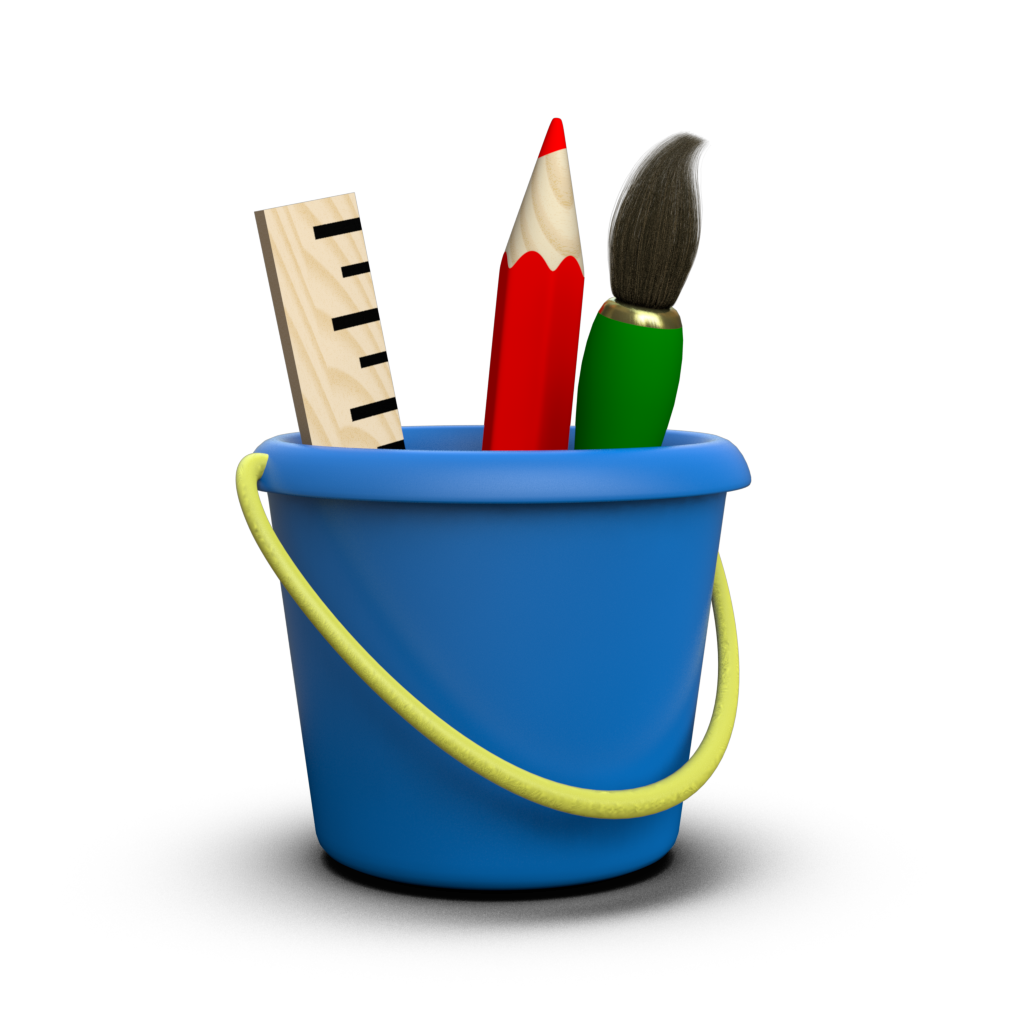# Units¶

There are several places in Toyplot where you will need to specify quantities with real-world units, including canvas dimensions, font sizes, and target dimensions for document-oriented backends such as toyplot.pdf. For example, when creating a canvas, whether explicitly or implicitly through the Convenience API, you could specify its width and height in inches:

:

import numpy
x = numpy.linspace(0, 1)
y = x ** 2

:

import toyplot
toyplot.plot(x, y, width="3in", height="2in");


You can also specify the quantity and units separately:

:

toyplot.plot(x, y, width=(3, "in"), height=(2, "in"));


If you rendered either plot using the PDF backend, the resulting document size would be 3 inches × 2 inches.

If you don’t specify any units, the canvas assumes a default unit of CSS pixels:

:

toyplot.plot(x, y, width=600, height=400);


Note: You’re probably used to treating pixels as dimensionless; however CSS Pixels are always 1/96th of an inch. Thus, the above example would produce a PDF document that is 6.25 inches × 4.16 inches.

If you rendered the same canvas using the PNG or MP4 backends, it would produce 600 × 400 pixel images / movies. Put another way, the backends that produce raster images always assume 96 DPI, unless overridden by the caller.

## Allowed Units¶

The units and abbreviations currently understood by Toyplot are as follows:

• centimeters - “cm”, “centimeter”, “centimeters”
• decimeters - “dm”, “decimeter”, “decimeters”
• inches - “in”, “inch”, “inches”
• meters - “m”, “meter”, “meters”
• millimeters - “mm”, “millimeter”, “millimeters”
• picas (1/6th of an inch) - “pc”, “pica”, “picas”
• pixels (1/96th of an inch) - “px”, “pixel”, “pixels”
• points (1/72nd of an inch) - “pt”, “point”, “points”

Functions that accept quantities with units as parameters will always accept them in either of two forms:

• A string that combines the value and unit abbreviations: “5in”, “12px”, “25.4mm”.
• A 2-tuple containing a number and string unit abbreviation: (5, “in”), (12, “px”), (25.4, “mm”).

In addition, some functions will also accept a single numeric value, with a documented default unit of measure (such as the canvas width and height discussed above).

Further, some functions may accept quantities with “%” as the units. In this case, the quantity will be relative to some other documented value.

## Style Units¶

Toyplot style parameters always explicitly follow the CSS standard. As such, they support a subset of unit abbreviations including “cm”, “in”, “mm”, “pc”, “px”, and “pt”. Although CSS provides additional units for relative dimensioning, they assume that the caller understands their relationship to the underlying Document Object Model (DOM). Because Toyplot does not expose the DOM to callers and may change it at any time, these units are not supported.